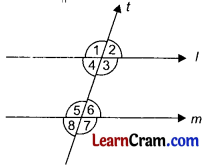# DAV Class 6 Maths Chapter 11 Brain Teasers Solutions

The DAV Maths Book Class 6 Solutions and DAV Class 6 Maths Chapter 11 Brain Teasers Solutions of Transversal and Pairs of Lines offer comprehensive answers to textbook questions.

## DAV Class 6 Maths Ch 11 Brain Teasers Solutions

Question 1.
A. Tick (✓) the correct answer.
(a) The opposite edges of a rectangular blackboard are:
(i) parallel
(ii) perpendicular
(iii) intersecting
(iv) none
(i) parallel.
Parallel lines do not intersect each other.

(b) In the given figure ‘t’ is a transversal to line l and m. The angles a and b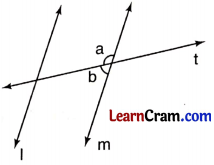(i) alternate angles
(ii) alternate interior angles
(iii) a linear pair
(iv) corresponding angles
(iii) linear pair.
Linear pairs are those angles which adding together to form an angle of 180°.

(c) A pair of corresponding angles in the given figures: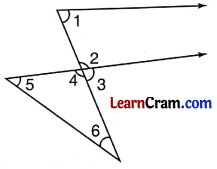(i) ∠1 and ∠6
(ii) ∠2 and ∠4
(iii) ∠3 and ∠4
(iv) ∠1 and ∠3
(iv) ∠1 and ∠3
Corresponding angles are on the same sides of the transversal but one of the angles is on the exterior sides of the given parallel lines. Here ∠1 is in the interior while ∠3 is in the exterior of the parallel lines.

(d) In the given figure, ‘t’ is a transversal to the lines AB and CD. ∠b and ∠h are: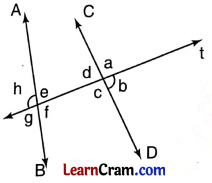(i) alternate interior angles
(ii) alternate exterior angles
(iii) corresponding angles
(iv) linear pair
(ii) alternate exterior angles
∠b and ∠h lie on the exterior of the parallel lines AB and CD but on the alternate sides of the transversal line ‘t’.

(e) In which figure given below, the angles marked are alternate angles?These angles lie on the alternate sides of the transversal but on the same side
i. e. interior side of the parallel lines.(a) Name any two pairs of parallel edges in the following cube.AB ∥ CG, AB ∥ HF, BC ∥ AG, BC ∥ HD, CG ∥ DE, AB ∥ DE, CG ∥ HF, BC ∥ EF, CD ∥ EG, BH ∥ CD, AF ∥ BH, AF ∥ CD, AF ∥ GE.
Name any two of them.

(b) Name all the transversal to the segments AC and FD.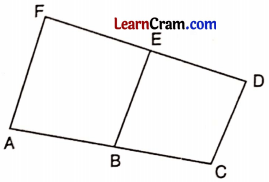AF, BE and CD.
These three lines are the transversal of the segments AC and FD.

(c) How many pairs of alternate angles are there?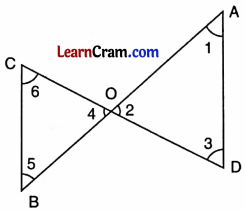Two pairs.
∠1 and ∠5 form one pair while ∠3 and ∠6 form another pair.

(d) From the given square, write any two pairs of perpendicular sides.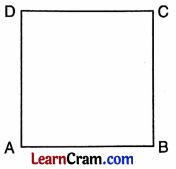AB ⊥ BC and AD ⊥ CD are two pairs. Another pairs are BC ⊥ CD and AB ⊥ AD.

(e) In the given figure, name the angle alternate to Z3.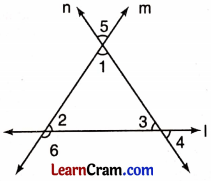∠3 and ∠6 are alternate interior angles.Question 2.
Identify parallel line segments in the figures given below:(a) AB ∥ CD
(b) AD ∥ EH ∥ FG ∥ BC

Question 3.
Identify alternate angles and corresponding angles from the given figure:(i) ∠2 and ∠3 are alternate angles,
(ii) ∠1 and ∠3, ∠2 and ∠4 are the pairs of corresponding angles.

Question 4.
Look at the figure and name the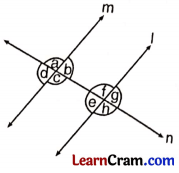(a) angle corresponding to ∠f
(b) angle alternate to ∠a
(c) angle alternate to ∠d
(d) angle corresponding to ∠c
(a) angle corresponding to ∠f = ∠a
(b) angle alternate to ∠a = ∠h
(c) angle alternate to ∠d = ∠g
(d) angle corresponding to ∠c = ∠h

Question 5.
In the given figure, name the angles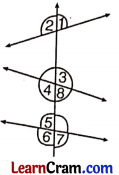(a) corresponding to:
(i) ∠2,
(ii) ∠4

(b) alternate to:
(i) ∠1
(ii) ∠5
(a) Angle corresponding to:
(i) ∠2 = ∠a
(ii) ∠4 = ∠6

(b) Angle alternate to:
(i) ∠1 = ∠4 or ∠6
(ii) ∠b = ∠8Question 1.
In the given figure, find: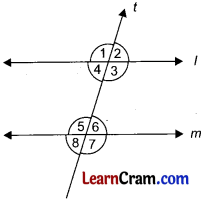(a) pair of interior alternate angles
(b) pair of exterior alternate angles
(c) pair of corresponding angles
(d) linear pairs
(a) ∠3 and ∠5, ∠4 and ∠6
(b) ∠1 and ∠7, ∠2 and ∠8
(c) ∠1 and ∠5, ∠2 and ∠6, ∠4 and ∠8, ∠3 and ∠7
(d) ∠1 and ∠2, ∠2 and ∠3, ∠3 and ∠4, ∠1 and ∠4, ∠5 and ∠6, ∠6 and ∠7, ∠7 and ∠8, ∠5 and ∠8

Question 2.
In the given figure l ∥ m and t is transversal. Find the value of x.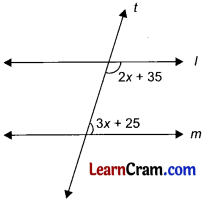(2x + 35)° + (3x + 25)° =180°
⇒ 2x + 35° + 3x + 25° =180°
(Sum of interior angles on the same side)
⇒ 5x + 60° =180°
⇒ 5x + 60° – 60° =180° – 60°
⇒ 5x =120°
x =120° ÷ 5 = 24

Question 3.
Identify the parallel line segments in the figure given below:AB ∥ CD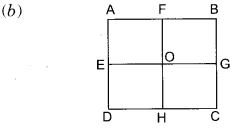AB ∥ EG ∥ DC, AD ∥ FH ∥ BC, AE ∥ FO ∥ BG, ED ∥ OH ∥ GC, AF ∥ EO ∥ DH, FB ∥ OG ∥ HC.AE ∥ FD, AF ∥ ED, FE ∥ BD, EF ∥ BC, FD ∥ AC, FD ∥ EC, ED ∥ AB, FE ∥ DC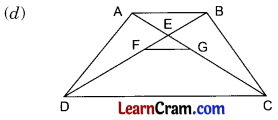AB ∥ FG ∥ DCAE ∥ BC